# COVID-19 Analysis: Uncertainties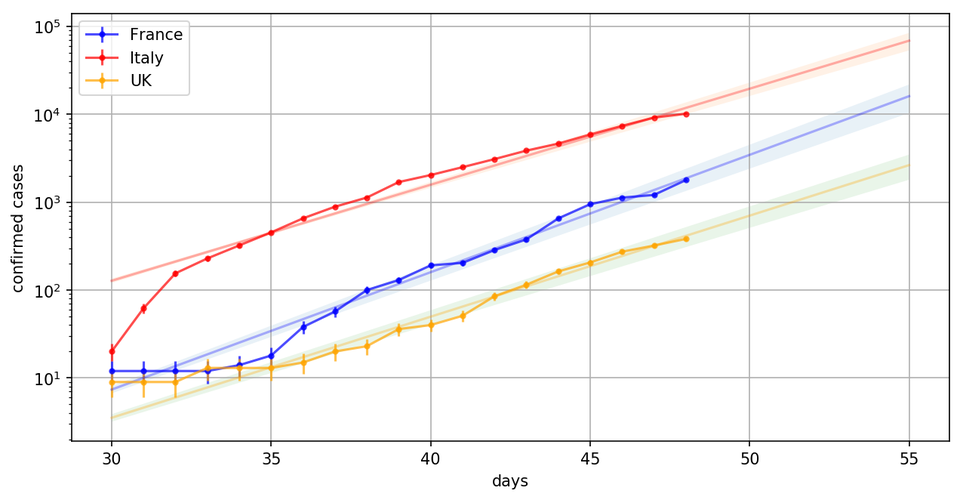## Introduction

In my previous post, COVID-19 Analysis, we have seen how to analyse the data provided by John Hopkins University with python. We have insisted on the technical aspects, such as data preprocessing with pandas and interactive visualization with holoviews, and we performed a simple exponential fit with scipy.optimize.

A caveat, however, is that we haven't considered uncertainties at all!

Here, you will learn:

• What are uncertainties and why we need them
• What is a covariance matrix
• How to propagate the uncertainties from the covariance matrix to compute an uncertainty band

## Data preparation¶

In :
import pandas as pd
datatemplate = 'csse_covid_19_data/csse_covid_19_time_series/time_series_19-covid-{}.csv'

fields = ['Confirmed', 'Deaths', 'Recovered']
dfs = dict()
for field in fields:

# loop on the dataframe dictionary
for field, df in dfs.items():
# group by country, to sum on states
df = df.groupby('Country/Region', as_index=False).sum()
# turn each measurement column into a separate line,
# and store the results in a new dataframe
df = df.melt(id_vars=['Country/Region', 'Lat', 'Long'],
value_name='counts')
# keep track of the quantity that is measured
# either Confirmed, Deaths, or Recovered
df['quantity'] = field
# change column names
df.columns =  ['country', 'lat', 'lon', 'date', 'counts', 'quantity']
# replace the dataframe in the dictionary
dfs[field] = df

dfall = pd.concat(dfs.values())
dfall['date'] = pd.to_datetime(dfall['date'])


## A Naive Fit¶

To get started, let's do a naive fit of the number of confirmed cases for France as a function of the number of days, as we did in the previous post.

• import the necessary tools and create the exponential function for the fit:
In :
import numpy as np
from scipy.optimize import curve_fit
import matplotlib.pyplot as plt

# exponential function for the fit
expo = lambda x, a, b : np.exp( a*(x-b) )

• prepare the data points to be fitted. We select the number of confirmed cases for France as y, and we prepare an array x ranging from 0 to the number of points in y:
In :
# select confirmed cases for France
sel = dfall[(dfall['country']=='France') &
(dfall['quantity']=='Confirmed')]

# y is the number of counts
yp = sel['counts']

# create x from 0 to number of points in yp
xp = np.arange(len(yp))

# fit the curve to get the parameters
pars, cov = curve_fit(expo, xp, yp)

• perform the fit to get the optimal parameters pars:
In :
# fit the curve to get the parameters
pars, cov = curve_fit(expo, xp, yp)

• plot the points and the fitted exponential. Note that the exponential is plotted in the same x range, but with higher granularity for a smoother curve.
In :
plt.scatter(xp, yp)
linx = np.linspace(0, xp.max(), 101)
f = expo(linx, *pars)
plt.plot(linx, f)

Out:
[<matplotlib.lines.Line2D at 0x11b645860>]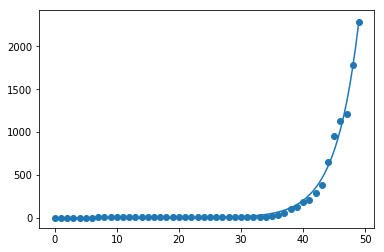Now we can extrapolate the function by simply extending the x range before plotting the fitted function:

In :
plt.scatter(xp, yp)
# extrapolate by 5 days:
linx = np.linspace(0, xp.max()+5, 101)
f = expo(linx, *pars)
plt.plot(linx, f)

Out:
[<matplotlib.lines.Line2D at 0x101e5f9630>]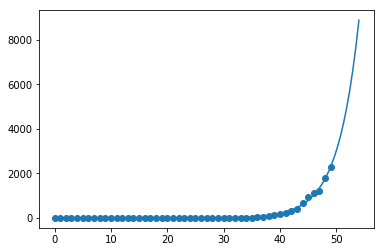And we can print the number of predicted cases:

In :
print('expected number of cases at day {} : {}'.format(
int(linx[-1]), int(f[-1])
))

expected number of cases at day 54 : 8868


## Why do We Need Uncertainties ?¶

But there is a big issue with this fit and with this prediction of the number of cases in the future: we did not take uncertainties into account!

For instance, let's imagine that the uncertainty in my prediction at day 53 is 50%. Then, we approximately have a 32% chance for the actual number of confirmed case to be either larger than 10 500, or smaller than 3500...

Assuming an uncertainty of 50% for the prediction of the model for all days, we can draw an uncertainty band:

In :
plt.scatter(xp, yp)
plt.plot(linx, f)
plt.fill_between(linx, f*(1+0.5), f*(1-0.5), alpha=0.2)

Out:
<matplotlib.collections.PolyCollection at 0x101e764a58>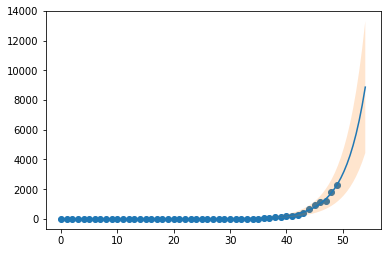That makes us a bit more cautious about interpreting the prediction, doesn't it?

In fact, in science, it is crucial to estimate the uncertainties properly. Just as important as predicting the central value.

Now, how can we estimate the uncertainties in our model?

Before we do that, we need to take a step back and think again about what we're trying to achieve with our fit.

There is a true law that describes the evolution of the epidemic as a function of time. This law determines what should be the number of cases as a function of time.

If we knew this law, we could predict perfectly the number of cases in the future.

But we don't... So the only thing we can do is to guess a model for this true function (here we went for an exponential, for the reasons explained in the previous post), and to fit the model to the measured points in order to extract the model parameters and model the law.

## Statistical Uncertainties¶

Let's say the true law predicts 10 cases on a given day.

This is a small number, so if you count the number of cases on that day, it is highly probable that you won't get exactly 10. Maybe you get 8 or 11.

Think about a coin. It has a 50-50 probability to give heads or tails. If you throw the coin 10 times, you're not going to always get 5-5.

So each count is affected by a statistical uncertainty that quantifies our ignorance of its true value. I don't want to go too much into details here, and it's enough to know that the uncertainty on a number of counts $n$ is

$$\Delta n = \sqrt{n}$$

Let's compute this uncertainty for all counts and display it:

In :
dn = np.sqrt(yp)
plt.errorbar(xp, yp, yerr=dn, ecolor='red')

Out:
<ErrorbarContainer object of 3 artists>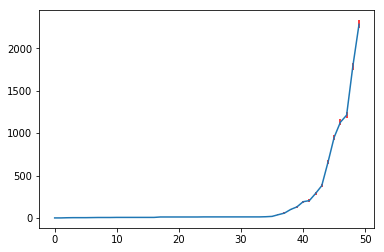We don't see much, so we redo the plot in log scale:

In :
plt.errorbar(xp, yp, yerr=dn, ecolor='red', marker='o')
plt.plot(linx, f)
plt.yscale('log')
plt.ylim(0.1, 3000)

Out:
(0.1, 3000)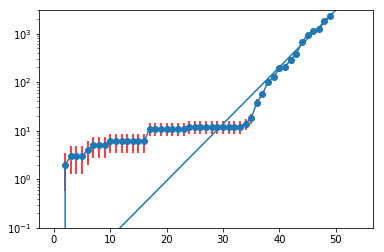This plot needs to be discussed:

• The first point has $n=2$, and so $\Delta n=\sqrt{2}$. This is what we see.
• The uncertainty bars show the range $\pm \Delta n$ around the central value $n$. They look asymmetric because of the log scale that compresses high values. But they are symmetric.
• The uncertainties for small counts look larger, again because of the log scale, but they are in fact smaller in absolute.
• The exponential model is a straight line, since
$${\rm ln}(e^{a(x-b)})=a(x-b)$$

The fit should take into account the uncertainties on the counts. Indeed, points with a small uncertainty should be allowed to strongly constrain the model, while points with a large uncertainty should have less weight.

The documentation of scipy.optimize.curve_fit tells us that if uncertainties are not provided as the sigma argument, all uncertainties are set to 1! this is clearly not what we want, so we redo the fit with the proper uncertainties:

In :
pars, cov = curve_fit(expo, xp, yp, sigma=dn)

/Users/cbernet/miniconda3/envs/covid19/lib/python3.6/site-packages/scipy/optimize/minpack.py:734: RuntimeWarning: divide by zero encountered in true_divide
transform = 1.0 / sigma
/Users/cbernet/miniconda3/envs/covid19/lib/python3.6/site-packages/scipy/optimize/minpack.py:808: OptimizeWarning: Covariance of the parameters could not be estimated
category=OptimizeWarning)


We get a division by zero error, because for some days at the beginning of the range, there was no confirmed case, hence $\Delta n=\sqrt{n}=0$. To fix this, we set the uncertainty to 1 for these days, which is more or less correct in the context of this problem... again I won't get into details, this is actually a rather philosophical question.

And we redo the fit, comparing with the previous one:

In :
dn[dn==0] = 1
# with uncertainties
pars, cov = curve_fit(expo, xp, yp, sigma=dn)
f2 = expo(linx, *pars)
plt.figure(figsize=(6,8))
# lin scale
plt.subplot(2,1,1)
plt.errorbar(xp, yp, yerr=dn, ecolor='red', marker='o')
plt.plot(linx, f, color='red', label='$\Delta N=1$')
plt.plot(linx, f2, color='blue', label='$\Delta N = \sqrt{N}$')
plt.legend()
plt.xlim(20, linx[-1])
# log scale
plt.subplot(2,1,2)
plt.errorbar(xp, yp, yerr=dn, ecolor='red', marker='o')
plt.plot(linx, f, color='red')
plt.plot(linx, f2, color='blue')
plt.ylim(1, 10000)
plt.xlim(20, linx[-1])
plt.yscale('log')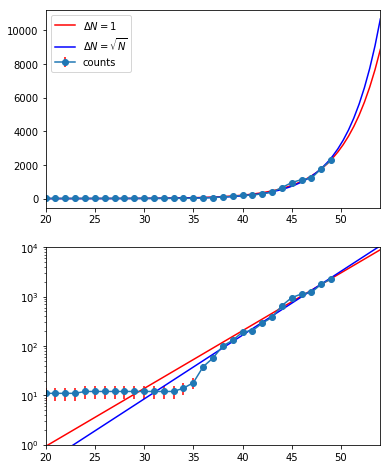Since the weight of the different points has changed, the fit converges to different parameters. And it matters a lot when you look at the difference in prediction between the two models.

But the statistical uncertainties do not matter only for the central value: the more uncertainty on each data point, the more uncertainty on the model parameters, and the larger the uncertainty band around the central value.

## The covariance matrix¶

We have seen that the fit returns two objects, pars and cov:

In :
pars, cov = curve_fit(expo, xp, yp, sigma=dn)
print(pars)
print(cov)

[ 0.29760344 22.8315399 ]
[[6.38094844e-05 4.99227264e-03]
[4.99227264e-03 3.99173828e-01]]


pars contains the optimal values $a_0$ and $b_0$ as estimated from the fit, and we already used these parameters to compute and display the central value for the fitted model.

cov is the covariance matrix, and we're now going to discuss it.

The fit is a minimization process. A cost function is minimized as a function of $a$ and $b$ and, when the minimum is reached, the corresponding (optimal) values for $a_0$ and $b_0$, are returned.

The covariance matrix is related to the shape of the cost function in the vicinity of the minimum.

Here is what the profile of the cost function looks like as a function of $a$ and $b$.

In :
from scipy.stats import multivariate_normal
from mpl_toolkits import mplot3d
import math
a0, b0 = pars
siga, sigb = np.sqrt(np.diag(cov))
linx = np.linspace(a0-3*siga, a0+3*siga, 201)
liny = np.linspace(b0-3*sigb, b0+3*sigb, 201)
x,y = np.meshgrid(linx,liny)
cost = - multivariate_normal.pdf(np.dstack([x,y]),
mean=pars,
cov=cov)
plt.contour(x,y,cost, zorder=0)
plt.scatter(a0, b0, marker='o', color='black',
s=100, zorder=2)
plt.scatter(a0+siga,b0+sigb, marker='o', color='red',
s=100, zorder=2)
plt.axvline(a0-siga, zorder=1)
plt.axvline(a0+siga, zorder=1)
plt.axhline(b0-sigb, zorder=1)
plt.axhline(b0+sigb, zorder=1)
plt.xlabel('a')
plt.ylabel('b')

Out:
Text(0, 0.5, 'b')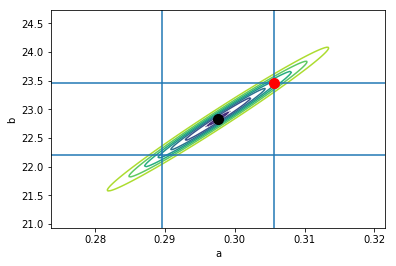The diagonal terms in the covariance matrix are the variances of $a$ and $b$. If we take the square root of these terms, we get the uncertainties on the $a$ and $b$ parameters, $\sigma_a$ and $\sigma_b$, as estimated by the fit from the shape of the cost function profile.

If we take the parameters independently, we see that $b$, the start of the epidemic, is determined with a precision of

In :
print('sigma_b = +- ', sigb)

sigma_b = +-  0.6318020482921778


a range indicated by the two horizontal lines in the plot. In the same way, we get for $a$ a precision of

In :
print('sigma_a = +- ', siga)

sigma_a = +-  0.007988083903202072


The off-diagonal term correspond to the covariance of the a and b parameters. The profile is tilted because of the non-zero covariance. This means that parameters a and b depend on each other.

I'd like to give you a feeling for this, because it's important.

From the profile of the cost function, we see that we have a flat dimension, the long axis of the ellipsis. If we move away from the central value along this dimension, the fit does not pay much price because the cost function increases very slowly along this dimension.

This means that the fit could very well have converged to a higher value of $b$, provided a higher value of $a$ is also chosen.

Why does it work this way? it's very easy to see by looking at the data points. If you increase $b$, the epidemic is delayed and the model curve is translated to the right without changing shape. Therefore, to be able to fit the data points that are now left to the curve, the fit needs to increase $a$ for a sharper rise.

Here is a comparison between the nominal model from the fit, and a model with $a=a_0 + \sigma_a$, and $b = b_0 + \sigma_b$ (indicated as a red point in the plot above):

In :
plt.errorbar(xp, yp, yerr=dn, ecolor='red', marker='o')
linx = np.linspace(0,50,101)
plt.plot(linx, expo(linx, *pars), color='blue')
plt.plot(linx, expo(linx, *(pars + np.sqrt(np.diag(cov)))),
color='red')
plt.yscale('log')
plt.ylim(0.1, 10000)
# plt.plot(linx, expo(linx, *(pars+np.diag(cov))))

Out:
(0.1, 10000)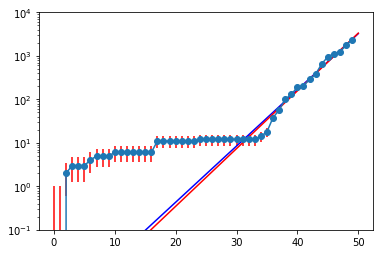## Calculating the uncertainty band¶

Ok, we understand the covariance matrix, and how it relates to the model uncertainty.

Now, we're going to use the covariance matrix to calculate the uncertainty band for the model.

To do this, we will propage the uncertainty from the model parameters to the model itself.

For any non-linear differentiable function $f(a,b)$, we have:

$$\sigma_f^2 = \left| \frac{\partial f}{\partial a} \right|^2 \sigma_a^2 + \left| \frac{\partial f}{\partial b} \right|^2 \sigma_b^2 + 2 \frac{\partial f}{\partial a}\frac{\partial f}{\partial b} \sigma_{ab}$$

Where $\sigma_f^2$ is the squared uncertainty on the value of the function, and $\sigma_a^2$, $\sigma_b^2$, and $\sigma_{ab}$ are the coefficients of the covariance matrix, which we have.

In our case,

$$f = e^{a(x-b)}$$

and we just need to compute the partial derivatives of this function with respect to $a$ and $b$. We have:

$$\frac{\partial f}{\partial a} = x f$$$$\frac{\partial f}{\partial b} = -a f$$

so:

$$\sigma_f^2 = f^2 (x^2\sigma_a^2 + a^2\sigma_b^2 - 2xa \sigma_{ab})$$

So let's code a little function to compute $\sigma_f$:

In :
def sigmaf(x, f, a0, cov):
sigmaa2 = cov[0,0]
sigmab2 = cov[1,1]
sigmaab = cov[0,1] # or 1,0
return f * np.sqrt(x**2 * sigmaa2 + a0**2 * sigmab2 - 2*x*a0*sigmaab)


We can now compute $\sigma_f$ for all values of $x$:

In :
linx = np.linspace(0,50,101)
f = expo(linx, *pars)
df = sigmaf(linx, f, pars, cov)


And finally we can plot the data points and the model with its uncertainty band:

In :
plt.errorbar(xp, yp, yerr=dn, ecolor='red', marker='o')
plt.plot(linx, f)
plt.fill_between(linx, f-df, f+df, alpha=0.2)
plt.xlim(30, 50)

Out:
(30, 50)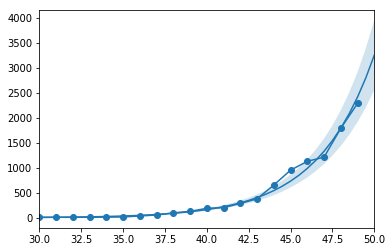## The function you've been waiting for¶

Finally, here is a function that you can use to plot any country:

In :
def plot(countries, xrange,
dtype='Confirmed',
yrange=None,
yscale='linear'):
'''plot the covid-19 data with an exponential fit.
- countries: list of countries
- xrange: fit range, e.g. (30,55)
- yscale: log or linear
- yrange: well, y range, useful in log mode.
'''
xmin, xmax = xrange
linx = np.linspace(xmin, xmax, 101)
colors = ['blue', 'red', 'orange', 'green']
for i, country in enumerate(countries):
color = colors[i]
sel = dfall[ (dfall['country']==country) &
(dfall['quantity']==dtype)]
yp = sel['counts'][xmin:xmax+1]
xp = np.arange(len(yp))+xmin
syp = np.sqrt(yp)
syp[syp==0]=1
plt.errorbar(xp, yp, yerr=syp, label=country,
alpha=0.7, marker='.', color=color)
pars, cov = curve_fit(expo, xp, yp, sigma=syp)
f = expo(linx, *pars)
plt.plot(linx, f,
color=color, alpha=0.3)
df = sigmaf(linx, f, pars, cov)
bandp = f+df
bandm = f-df
plt.fill_between(linx, bandm, bandp, alpha=0.1)
plt.legend(loc='upper left')
plt.xlabel('days')
plt.ylabel('confirmed cases')
plt.yscale(yscale)
if yrange:
plt.ylim(*yrange)
plt.grid()

plt.figure(dpi=150, figsize=(10,5))
plot(['France', 'Italy', 'Switzerland'],
dtype='Confirmed',
xrange=(30, 55),
yscale='log')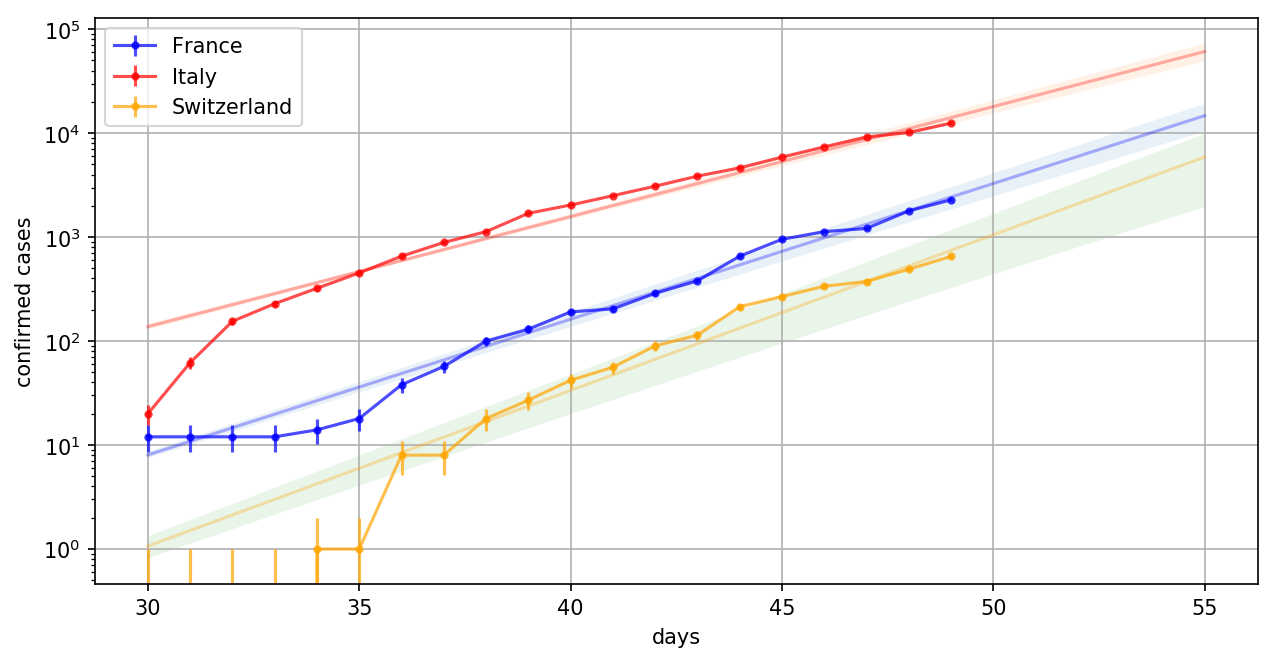## More Caveats

Kyle Cranmer kindly pointed out that I am currently assuming the counts on each day to be uncorrelated, while they are. This makes the fit look too good.

That's absolutely true. Indeed, the counts at day $d+1$ include the counts at day $d$. So if there is any fluctuation at day $d$, we'll get a remnant of this fluctuation at day $d+1$.

Clearly, this should be taken into account, but I don't really know how to do this. Usually, I make sure to fit uncorrelated data points so that I don't have to think about this :-)

Anyway, I think that the fit can still be somehow trusted because:

• We have a fast rising function, so that on day $d+1$, we have a fair amount of new points.
• The statistical uncertainties on each count are actually small. Most of the fit uncertainties come from the fact that we try to fit an exponential model to data points that do not really follow an exponential.

All of this shows that uncertainties are not easy. You should be careful and really know what you're doing. And I hope I got it right... !

Patrick Janot also made an important comment. The number of confirmed cases is not the number of infected people, just the number of people tested positive.

This means that if we stop testing for some reason (too many potential cases, not enough test kits, obvious nature of the disease), we'll see an inflexion in the number of confirmed cases, making us think that we are able to stop the epidemic. It could be that the number of infected is already much larger than the number of confirmed cases, especially if many people only have mild symptoms.

All of this is very well explained in this article: Coronavirus: Why You Must Act Now. I strongly encourage you to read it.

## Conclusion

We can predict that France will reach the status of Italy today in just 5 days, if nothing changes.

There is therefore a high probability for the country to get completely blocked in the following couple days. French people, don't plan too much for next week.

And my opinion is that these numbers should be in the hands of the government already. I guess the only reason why strong restrictive measures have not yet been taken is that the country is not ready to accept them before we reach the symbolic threshold of 10,000 confirmed cases.

Please do take the recommendations seriously, for the sake of older people, and of people with a chronic health situation.